# The Chemistry of Acids and BasesPage 3

#### WATCH ALL SLIDES

Slide 24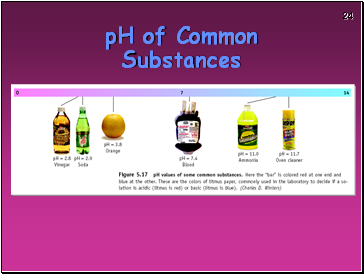pH of Common Substances

Slide 25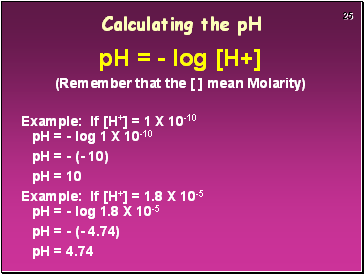Calculating the pH

pH = - log [H+]

(Remember that the [ ] mean Molarity)

Example: If [H+] = 1 X 10-10 pH = - log 1 X 10-10

pH = - (- 10)

pH = 10

Example: If [H+] = 1.8 X 10-5 pH = - log 1.8 X 10-5

pH = - (- 4.74)

pH = 4.74

Slide 26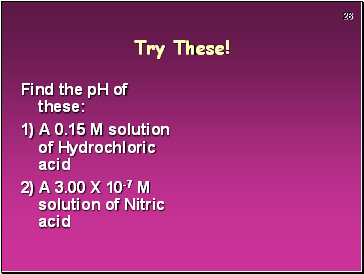Try These!

Find the pH of these:

1) A 0.15 M solution of Hydrochloric acid

2) A 3.00 X 10-7 M solution of Nitric acid

Slide 27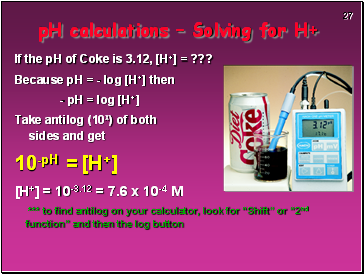pH calculations – Solving for H+

If the pH of Coke is 3.12, [H+] = ???

Because pH = - log [H+] then

- pH = log [H+]

Take antilog (10x) of both sides and get

10-pH = [H+]

[H+] = 10-3.12 = 7.6 x 10-4 M

*** to find antilog on your calculator, look for “Shift” or “2nd function” and then the log button

Slide 28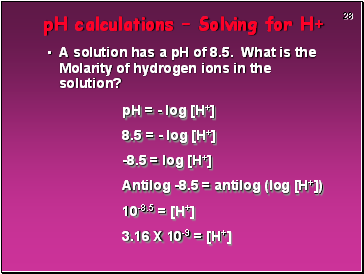pH calculations – Solving for H+

A solution has a pH of 8.5. What is the Molarity of hydrogen ions in the solution?

pH = - log [H+]

8.5 = - log [H+]

-8.5 = log [H+]

Antilog -8.5 = antilog (log [H+])

10-8.5 = [H+]

3.16 X 10-9 = [H+]

Slide 29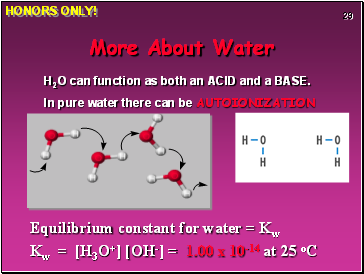H2O can function as both an ACID and a BASE.

In pure water there can be AUTOIONIZATION

Equilibrium constant for water = Kw

Kw = [H3O+] [OH-] = 1.00 x 10-14 at 25 oC

HONORS ONLY!

Slide 30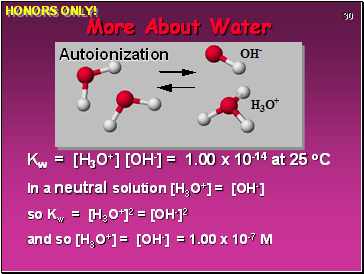Kw = [H3O+] [OH-] = 1.00 x 10-14 at 25 oC

In a neutral solution [H3O+] = [OH-]

so Kw = [H3O+]2 = [OH-]2

and so [H3O+] = [OH-] = 1.00 x 10-7 M

Autoionization

HONORS ONLY!

Slide 31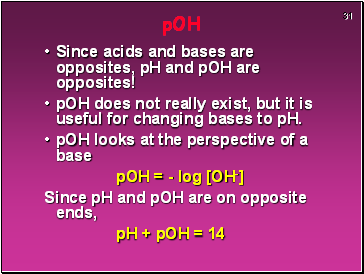pOH

Since acids and bases are opposites, pH and pOH are opposites!

pOH does not really exist, but it is useful for changing bases to pH.

pOH looks at the perspective of a base

pOH = - log [OH-]

Since pH and pOH are on opposite ends,

pH + pOH = 14

Slide 32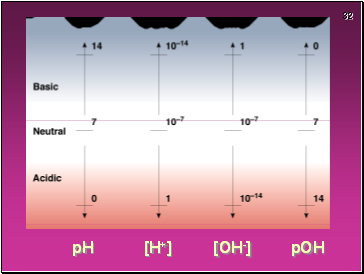pH

[H+]

[OH-]

pOH

Slide 33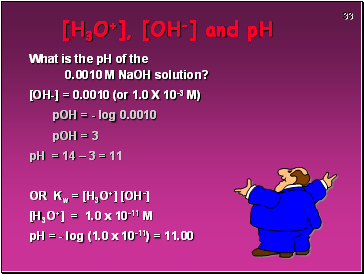[H3O+], [OH-] and pH

What is the pH of the 0.0010 M NaOH solution?

[OH-] = 0.0010 (or 1.0 X 10-3 M)

pOH = - log 0.0010

pOH = 3

pH = 14 – 3 = 11

OR Kw = [H3O+] [OH-]

[H3O+] = 1.0 x 10-11 M

Go to page:
1  2  3  4  5  6  7  8